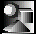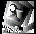# Introduction to Scientific Programming:

## Author

Joseph L. Zachary
Department of Computer Science
University of Utah

## Overview

Introduction to Scientific Programming was developed over a period of three years for use in teaching a course in scientific computing to beginning science and engineering students at the University of Utah.

In conventional science and engineering degree programs, computation is commonly treated as a narrow technical tool to be studied and applied in isolation from the traditional topics of the discipline. Many students go through their degree programs without ever using computation in any significant way.

Introduction to Scientific Programming was designed to encourage the integration of computation into the science and engineering curricula. This textbook is ideal for a course whose goal is to teach introductory programming while simultaneously preparing students to immediately exploit the broad power of modern computing in their science and engineering courses. This textbook has at least four distinguishing features:

• A symbolic mathematics package (Maple) is used in combination with a conventional programming language (C).
• Programming concepts are taught in parallel with a computational science problem-solving methodology.
• A variety of computational problems are used from the breadth of science and engineering to interest students and establish the relevance of the computational problem-solving approach.
• An extensive suite of interactive, on-line laboratory materials have been developed. These include HTML-based tutorials enhanced with Java applets, Maple worksheets, and C programs.

## Contents

Introduction to Scientific Programming is organized into a series of short, focused chapters. Each chapter begins by introducing a problem and then guiding the student through its solution. The computational techniques needed to solve the problem are developed as necessary. In this way, the motivation for learning the computing is always apparent. This deliberate problem-orientation is one of the defining characteristics of this book. In most other books that claim to be problem-oriented the examples are typically presented after the computing.

Understanding the computational problem-solving process is at least as important as understanding how to write code. Each of the chapters focuses on solving the motivating problem via the following approach:Problem. Clearly and unambiguously identify the problem to be solved.Model. Use simplification and abstraction to arrive at a mathematical model for the problem.Method. Identify a computational method for solving the model.Implementation. Implement the method in the appropriate language.Assessment. Concurrently with the other four steps, actively assess the problem-solving process to identify mistakes and unwarranted assumptions.

1. Computational Science
Chapter 1 explores the differences among the experimental, theoretical, and computational approaches to science, using the work of Tycho Brahe, Isaac Newton, and Johannes Kepler as motivating examples.

Programming in Maple
Chapters 2--9 explore programming in the context of Maple. The focus is on the computational properties of numbers, arithmetic and symbolic expressions, programmer-defined functions, and scientific visualization. Chapter 9 introduces procedural, statement-oriented programming to prepare students for the transition to C.

2. Population Density: Computational Properties of Numbers
An introduction to Maple using the problem of determining the average population density of the earth as the starting point for learning how to use rational numbers, floating-point numbers, and arithmetic expressions to do simple calculations.

3. Eratosthenes: Significant Digits and Interval Arithmetic
A simple experiment and geometric argument that Eratosthenes of Cyrene used to determine the circumference of the earth in 225 BC is used to explore the methods of significant digits and interval arithmetic.

4. Stairway to Heaven: Accumulation of Roundoff Error
This chapter uses the amusing problem of determining how far beyond the edge of a table an overhanging stack of blocks can be made to extend to explore the tradeoffs between rational and floating-point arithmetic.

5. Kitty Hawk: Programmer-Defined Functions
The problem of determining the line-of-sight distance to the horizon from the top of a hill leads to a comparison of two algebraically identical but computationally distinct methods and to an introduction to programmer-defined functions.

6. Baby Boom: Symbolic Computation
This chapter exploits the problem of predicting the population growth of the United States as an opportunity to branch out from the numerical focus of earlier chapters to examine symbolic programming.

7. Ballistic Trajectories: Scientific Visualization
The problem of visualizing the ballisitic trajectory of a projectile is used to explore Maple's two-dimensional plotting and animation capabilities.

8. The Battle for Leyte Gulf: Symbolic Expression Manipulation
Chapter 8 explores the problem of computing and visualizing the power required to move a ship along a specified one-dimensional trajectory through the water.

9. Old MacDonald's Cow: Procedural Programming
The problem of finding the radius of an arc that divides a circle into equal areas is used to motivate the bisection method, whose implementation provides an introduction to procedural programming.

Programming in C
Chapters 10--18 explore programming in the context of C. The book builds on the concepts introduced in the context of Maple to explore ideas from statement-oriented procedural programming, including assignment, input/output, conditionals, programmer-defined functions, loops, structures, and arrays.

10. Introduction to C
This chapter marks the transition point between the treatments of Maple and C. Statement-oriented procedural programming in C is introduced and the differences in programming in Maple and C are outlined.

11. Robotic Weightlifting: Straight-Line Programs
The problem of controlling the ankle, knee, and hip joints of a robot doing a squat exercise is explored. A C implementation of the model leads to a discussion of straight-line programs that touches on numerical types, expressions, assignment statements, the C math library and the C input/output library.

12. Sliding Blocks: Conditionals
The problem of calculating the motion of a block on an inclined ramp is used to explore the variants of the C if statement. Function headers, function bodies, function prototypes, and runtime behavior of functions are also explored.

13. Rod Stacking: Designing with Programmer-Defined Functions
The problem of determining the center coordinates of each rod in a stack of cylindrical rods is used to illustrate the role of functional decomposition in program design, implementation, and testing.

14. Newton's Beam: Repetition
Newton's method is introduced in order to determine the positioning of a beam that is laid against a cubical box. The workings of while and do loops in C are explained.

15. Numerical Integration: Multiple-File Programs
Methods of numerical integration are introduced to determine the length of a steel sheet that is to be 10 meters long after being corrugated. for loops, passing functions as parameters, and the advantages of dividing large programs into multiple files in C are discussed.

16. Harmonic Oscillation: Structures and Abstract Datatypes
To solve the problem of determining the position, as a function of time, of a block undergoing damped harmonic oscillation, Newton's method is extended to the complex domain. This leads to a discussion of structures and abstract datatypes in C.

17. Heat Transfer in a Rod: Arrays
Determining the temperature, as a function of time, at the center of a silver rod after heat sources have been applied at its ends leads to a finite-element model whose computational solution requires the use of arrays.

18. Visualizing Heat Transfer: Arrarys as Parameters
The chapter addresses the problem of visualizing the temperature of the rod from the previous chapter as it undergoes heating. A C program is used to create a Maple program that will display the desired animation.

Appendix A: Maple Built-In Functions
Appendix B: C Library Functions
Bibliography
Index
1996/380 pages/Hardcover
ISBN 0-387-94630-6

## Online Resources

An extensive suite of online educational resources has been (and continues to be) developed to support courses that use Introduction to Scientific Programming. These resources include HTML-based tutorials that can be viewed with any Java-capable Web browser, custom Maple libraries, Maple worksheets, and C programs.

Joseph L. Zachary is an Associate Professor of Computer Science at the University of Utah where he does research into the application to education of computing, connectivity, and communications. He received a Ph.D. in Computer Science from Massachusetts Institute of Technology in 1987. He has received numerous teaching awards from the University of Utah and has developed Internet-based software and course material that have been used to teach introductory computer science courses to thousands of students since 1991.

## To Order

• Detailed Ordering Information

• To order by regular mail, send orders to:
Springer-Verlag New York, Inc.
PO Box 2485
Secaucus, NJ 07096-2485

• To order by phone, call: 1-800-777-4643

• To order by fax: 201-348-4505